Next: Shifts and phase changes Up: Properties of Fourier transforms Previous: Properties of Fourier transforms   Contents   Index

## Fourier transform of DC

Let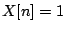for all(this repeats with any desired integer period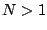). From the preceding discussion, we expect to find thatWe will often need to know the answer for non-integer values ofhowever, and for this there is nothing better to do than to calculate the value directly: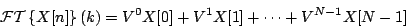whereis, as before, the unit magnitude complex number with argument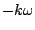. This is a geometric series; as long aswe get:We now symmetrize the top and bottom in the same way as we earlier did in Section 7.3. To do this let:so that. Then factoring appropriate powers ofout of the numerator and denominator gives: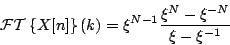It's easy now to simplify the numerator:and similarly for the denominator, giving: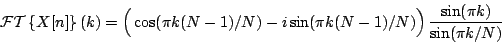Whetheror not, we havewhere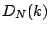, known as the Dirichlet kernel, is defined as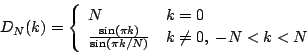Figure 9.1 shows the Fourier transform of, with. The transform repeats every 100 samples, with a peak at, another at, and so on. The figure endeavors to show both the magnitude and phase behavior using a 3-dimensional graph projected onto the page. The phase termacts to twist the values of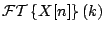around theaxis with a period of approximately two. The Dirichlet kernel, shown in Figure 9.2, controls the magnitude of. It has a peak, two units wide, around. This is surrounded by one-unit-wide sidelobes, alternating in sign and gradually decreasing in magnitude asincreases or decreases away from zero. The phase term rotates by almost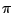radians each time the Dirichlet kernel changes sign, so that the product of the two stays roughly in the same complex half-plane for(and in the opposite half-plane for). The phase rotates by almost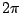radians over the peak from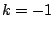to.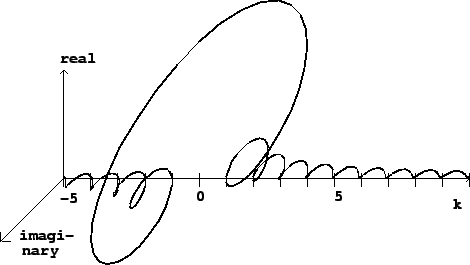Next: Shifts and phase changes Up: Properties of Fourier transforms Previous: Properties of Fourier transforms   Contents   Index
Miller Puckette 2006-12-30